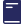Over 1000 Titles for students & teachers

# Excel Year 11 & 12 Mathematics Extension 1

New
\$39.95

#### About This Book

This brand new full-colour format has even more features to help students succeed in Year 11 and 12 Mathematics Extension 1.

YEAR 11

Topic: Functions

Chapter 1: Further work with functions

Chapter 2: Polynomials

Topic: Trigonometric functions

Chapter 3: Further trigonometric identities

Chapter 4: Inverse trigonometric functions

Topic: Calculus

Chapter 5: Rates of change

Topic: Combinatorics

Chapter 6: Working with combinatorics

Sample Year 11 examination paper

Answers

Year 12

Topic: Proof

Chapter 8: Proof by mathematical induction

Topic: Vectors

Chapter 9: Introduction to vectors

Chapter 10: Further operations with vectors

Chapter 11: Projectile motion

Topic: Trigonometric functions

Chapter 12: Trigonometric equations

Topic: Calculus

Chapter 13: Further calculus skills

Chapter 14: Further area and volumes of solids of revolution

Chapter 15: Differential equations

Topic: Statistical analysis

Chapter 16: The binomial distribution

Index

Sample HSC Examination papers

Answers

Index

Reference sheet

#### Product Details

SKU:
9781741256833
YEAR:
YEAR:
Year 11
Year 12
ISBN:
9781741256833
AUTHOR:
Allyn Jones
BRAND:
Excel
SUB BRAND:
Excel Year 11 & 12
SUBJECT:
Mathematics Extension 1
TYPE:
Study Guides